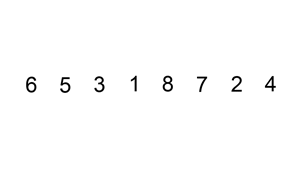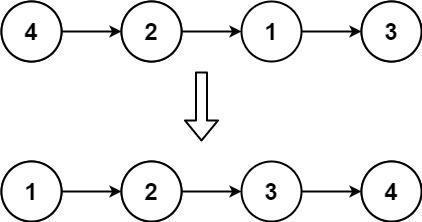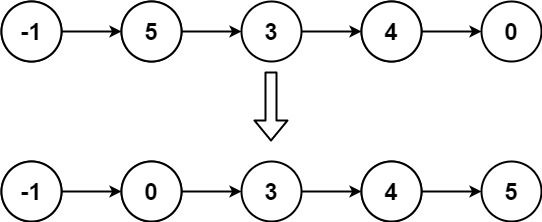# Question

Formatted question description: https://leetcode.ca/all/147.html

Given the head of a singly linked list, sort the list using insertion sort, and return the sorted list's head.

The steps of the insertion sort algorithm:

1. Insertion sort iterates, consuming one input element each repetition and growing a sorted output list.
2. At each iteration, insertion sort removes one element from the input data, finds the location it belongs within the sorted list and inserts it there.
3. It repeats until no input elements remain.

The following is a graphical example of the insertion sort algorithm. The partially sorted list (black) initially contains only the first element in the list. One element (red) is removed from the input data and inserted in-place into the sorted list with each iteration.Example 1:Input: head = [4,2,1,3]
Output: [1,2,3,4]


Example 2:Input: head = [-1,5,3,4,0]
Output: [-1,0,3,4,5]


Constraints:

• The number of nodes in the list is in the range [1, 5000].
• -5000 <= Node.val <= 5000

# Algorithm

One by one element is taken out of the original linked list and then inserted into the new linked list in order.

The time complexity is O(n^2), which is an algorithm that is not very efficient, but the space complexity is O(1), in exchange for high time complexity for low space complexity.

# Code

• 
public class Insertion_Sort_List {

/**
* public class ListNode {
*     int val;
*     ListNode next;
*     ListNode(int x) { val = x; }
* }
*/
public class Solution {

return null;
}

// for current tail, maintain its prev and itself, for moving around
ListNode dummy = new ListNode(0);
// dummy.next = head; // @note:@memorize: here is the start of wrong

/*
@note: I think the key is to cut the list into 2 half, un-linked
I was trying to move node around using i, j to indicate the progress of sorted part, like insert-sort in arrays
but it was nightmare moving pointers

the key is the same as the below solution which create new nodes
but here no need to create new nodes, just pointer moving
and key is the same, keep 2 separate, un-linked, un-related lists
*/

while (current != null) {
// every time, scanner will scan from begging of sorted list, to find where to insert
ListNode scanner = dummy;

// @note:@memorize: here use scanner.next, so that insert postion is at scanner next
while (scanner.next != null && scanner.next.val < current.val) {
scanner = scanner.next;
}

// record for next loop
ListNode nextRoundStart = current.next;

// insert
current.next = scanner.next;
scanner.next = current;

// update
current = nextRoundStart;

}

return dummy.next;
}

}

// create a separate list, extra space...
public class Solution_extra_space {

ListNode dummy = new ListNode(0);

while(current != null) {

ListNode prev = dummy;
ListNode newnode = new ListNode(current.val);

while(prev.next != null && newnode.val > prev.next.val) {
prev = prev.next;
}

// insert
ListNode oldPrevNext = prev.next;
prev.next = newnode;
newnode.next = oldPrevNext;

current = current.next; // loop through the list
}

return dummy.next;
}
}

}

############

/**
* public class ListNode {
*     int val;
*     ListNode next;
*     ListNode() {}
*     ListNode(int val) { this.val = val; }
*     ListNode(int val, ListNode next) { this.val = val; this.next = next; }
* }
*/
class Solution {
}
ListNode pre = dummy, cur = head;
while (cur != null) {
if (pre.val <= cur.val) {
pre = cur;
cur = cur.next;
continue;
}
ListNode p = dummy;
while (p.next.val <= cur.val) {
p = p.next;
}
ListNode t = cur.next;
cur.next = p.next;
p.next = cur;
pre.next = t;
cur = t;
}
return dummy.next;
}
}

• // OJ: https://leetcode.com/problems/insertion-sort-list/
// Time: O(N^2)
// Space: O(1)
class Solution {
public:
ListNode dummy;
auto p = &dummy;
while (p->next && p->next->val < node->val) p = p->next;
node->next = p->next;
p->next = node;
}
return dummy.next;
}
};

• # Definition for singly-linked list.
# class ListNode:
#     def __init__(self, val=0, next=None):
#         self.val = val
#         self.next = next
class Solution:
def insertionSortList(self, head: ListNode) -> ListNode:
while cur:
if pre.val <= cur.val:
pre, cur = cur, cur.next
continue
p = dummy
while p.next.val <= cur.val:
p = p.next
t = cur.next
cur.next = p.next
p.next = cur
pre.next = t
cur = t
return dummy.next

############

class Solution(object):
p = dummy = ListNode(0)
while cur and cur.next:
val = cur.next.val
if cur.val < val:
cur = cur.next
continue
if p.next.val > val:
p = dummy
while p.next.val < val:
p = p.next
new = cur.next
cur.next = new.next
new.next = p.next
p.next = new
return dummy.next


• /**
* function ListNode(val, next) {
*     this.val = (val===undefined ? 0 : val)
*     this.next = (next===undefined ? null : next)
* }
*/
/**
* @return {ListNode}
*/
var insertionSortList = function (head) {
let prev = dummy,
while (cur != null) {
if (prev.val <= cur.val) {
prev = cur;
cur = cur.next;
continue;
}
let p = dummy;
while (p.next.val <= cur.val) {
p = p.next;
}
let t = cur.next;
cur.next = p.next;
p.next = cur;
prev.next = t;
cur = t;
}
return dummy.next;
};